# nLab all horizontal weight systems are partitioned Lie algebra weight systems

Contents

### Context

#### Knot theory

knot theory

Examples/classes:

Types

knot invariants

link invariants

Related concepts:

category: knot theory

# Contents

## Idea

All weight systems on horizontal chord diagrams may be realized as linear combinations of Lie algebra weight systems applied not necessarily to the given horizontal chord diagram itself, but to the result of regarding each of its strands as resolved by some finite number of strands.

We state this precisely as Prop. below (due to Bar-Natan 96). First we introduce all the definitions that enter the statement:

## Ingredients

Given any ground field $\mathbb{F}$ (or in fact just any commutative ground ring)

1) write $\mathcal{A}^{pb}$ for the linear span of horizontal chord diagrams modulo the 2T relations and the 4T relations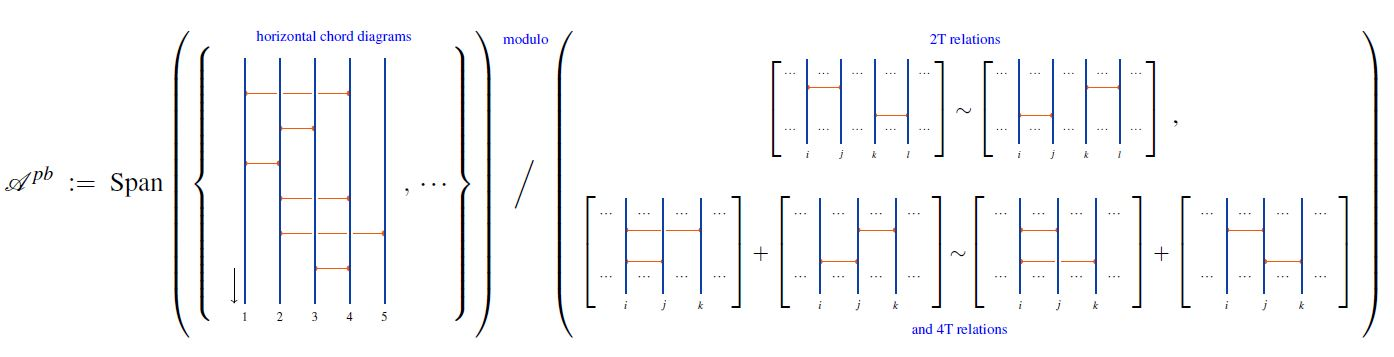graphics from Sati-Schreiber 19c

regarded as a graded vector space, graded by the number of chords,

and write

(1)$\mathcal{W}_{pb} \coloneqq (\mathcal{A}^{pb})^\ast$

for its degreewise dual vector space: the space of horizontal weight systems;

2) write

$\mathcal{A}^{pb} \overset{ \;\;\; p_n \;\;\; }{\longrightarrow} \mathcal{A}^{pb}_n \overset{ \;\;\; i_n \;\;\; }{\hookrightarrow} \mathcal{A}^{pb}$

for projection onto and inclusion of the linear subspace spanned by horizontal chord diagrams with $n$ strands;

3) write

$\underset{\mathbb{N}}{\oplus} \mathbb{N} \overset{ \;\;\; \Delta^{(-)} \;\;\; }{\longrightarrow} End( \mathcal{A}^{pb} \big)$

for the operation that reads in a finite tuple $k \coloneqq (k_1, \cdots, k_n)$ of natural numbers, with sum $\left\vert k\right\vert \coloneqq \underset{i}{sum} k_i$, and produces the linear map

(2)$\mathcal{A}^{pb} \overset{ \; p_n \; }{\to} \mathcal{A}^{pb}_{n} \overset{ \;\;\; \Delta^k \;\;\; }{\longrightarrow} \mathcal{A}^{pb}_{\left\vert k \right \vert} \overset{ \; i_{\left\vert k \right\vert} \; }{\hookrightarrow} \mathcal{A}^{pb}$

which takes a horizontal chord diagram with $n$ strands to the linear combination of chord diagrams obtained by replacing its $i$-th strand by $k_i$ strands for all $i$ and then summing over all ways of re-attaching chords, with any vertex previously on some strand $i$ now to be put on one of the $k_i$ strands (Bar-Natan 96, Def. 2.2).

For example: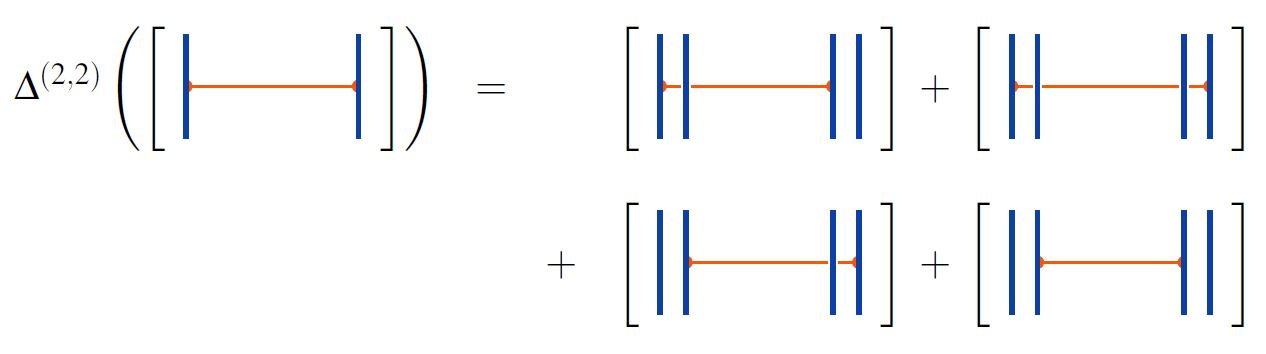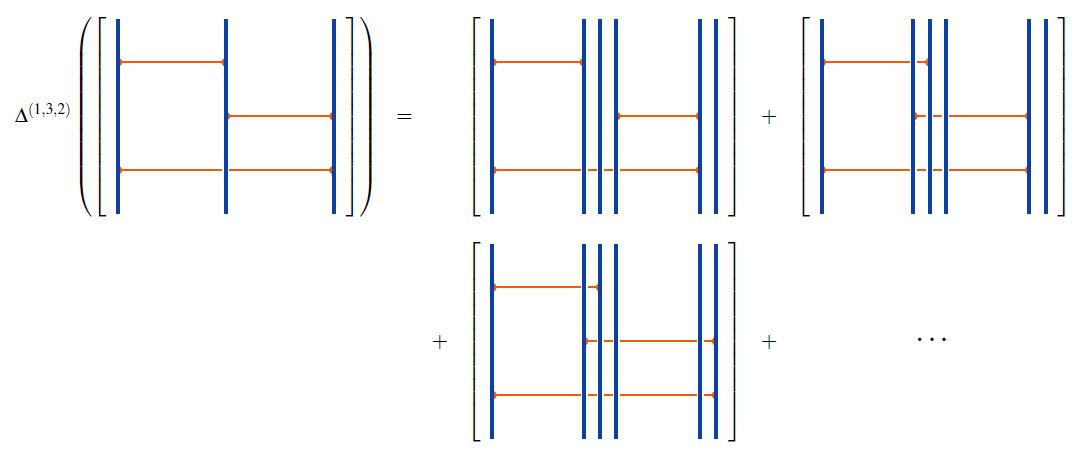graphics from Sati-Schreiber 19c

Moreover, for $\mathfrak{g}$ a metric Lie algebra

1) write

$\mathfrak{g}Mod_{/\sim}$

2) write

(3)$\mathfrak{g}Mod_{/\sim} \overset{ w_{(-)} }{\longrightarrow} \underset{n \in \mathbb{N}}{\oplus} Hom_{\mathbb{F}} \big( \mathcal{A}^{pb}_n , End(C^{\otimes n}) \big)$

for the function that sends a Lie module $C$ over $\mathfrak{g}$ to the corresponding endomorphism ring-valued Lie algebra weight system $w_C$ on horizontal chord diagrams.

Finally, for

1. $C \in \mathfrak{g} Mod$ a Lie algebra representation of $\mathfrak{g}$,

2. $n \in \mathbb{N}$ a natural number,

3. $\sigma \in Sym(n)$ a permutation of $n$ elements

write

(4)$tr_\sigma \;\colon\; End \big( C^{\otimes n} \big) \longrightarrow \mathbb{F}$

for the composite operation of

1. composing an endomorphism on the $n$-fold tensor power of $C$ by the braiding according to the permutation $\sigma$;

2. forming the trace of the resulting endomorphism of $C^{\otimes n}$.

Then the composition of

1. the partitioning function (2);

2. the assignment (3) of Lie algebra weight systems;

3. the permuted trace operation (4)

yields a function from triples consisting of a Lie module, a tuple of natural numbers and a permutation to horizontal weight systems:

(5)$\array{ \big( \mathfrak{g}Mod_{/\sim} \big) \;\times\; \big( \underset{\mathbb{N}}{\oplus} \mathbb{N} \big) \; \underset{ \mathbb{N} }{\times} \; \big( \underset{n \in \mathbb{N}}{\sqcup} Sym(n) \big) & \overset{ \;\; tr_{(-)} \circ w_{(-)} \circ \Delta \;\; }{ \longrightarrow } & \mathcal{W}_{pb} \\ (C, \; k = (k_1, \cdots, k_n), \; \sigma) &\mapsto& \left( D \;\mapsto\; tr_\sigma \circ w_C \circ \Delta^k (D) \right) }$

Finally, write also

(6)$Span \big( \mathfrak{g}Mod_{/\sim} \;\times\; \underset{\mathbb{N}}{\oplus} \mathbb{N} \;\underset{\mathbb{N}}{\times}\; \underset{n \in \mathbb{N}}{\mathbb{N}} Sym(n) \big) \overset{ tr_{(-)} \circ w_{(-)} \circ \Delta^{(-)} (-) }{ \longrightarrow } \mathcal{W}_{pb}$

for the linear extension of this function (5) to the linear span of its domain set.

## Statement

###### Proposition

For $N \geq 2$ consider the special linear Lie algebra $\mathfrak{sl}(N)$, canonically regarded as a metric Lie algebra via its Killing form.

Then the space $\mathcal{W}_{pb} \coloneqq (\mathcal{A}^{pb})^\ast$ (1) of weight systems on horizontal chord diagrams is spanned by partitioned $\mathfrak{sl}(N)$-Lie algebra weight systems, in that the linear extension (6) of the function (5) assigning $\mathfrak{sl}(N)$-Lie algebra weight systems composed with partitioning (2) is an epimorphism:

$\array{ Span \Big( \big( \underset{ \mathclap{ \color{blue} {Lie\;modules} } }{ \underbrace{ \mathclap{\phantom{\vert \atop \vert}} \mathfrak{sl}(N) \, Mod_{/\sim} } } \big) \; \times \; \big( \underset{ \mathclap{ \color{blue} tuples\;of\;numbers } }{ \underbrace{ \mathclap{ \phantom{\vert \atop \vert } } \underset{\mathbb{N}}{\oplus} \mathbb{N} } } \big) \; \underset{\mathbb{N}}{\times} \; \big( \underset{ \color{blue} permutations }{ \underbrace{ \underset{n \in \mathbb{N}}{\sqcup} Sym(n) } } \big) \Big) & \underoverset{\color{blue}epimorphism}{ \;\;\; tr_{(-)} \circ w_{(-)} \circ \Delta^{(-)} (-) \;\;\; }{\longrightarrow} & \overset{ \mathclap{ {\color{blue} horizontal\;weight\;systems} \atop {\phantom{a}} } }{ \mathcal{W}_{pb} } \\ ( C, \;\; k = (k_1, \cdots, k_n), \;\; \sigma ) &\mapsto& \left( \;\;\;\;\;\; \array{ \overset{ \mathclap{ \color{blue} { {horizontal} \atop { {chord} \atop {diagram} } } \atop {\phantom{a}} } }{ D } \mapsto & \phantom{=\;} \overset{ \mathclap{ \color{blue} \sigma\text{-}trace } }{ \overbrace{ tr_\sigma } } \circ \underset{ \mathclap{ {\color{blue}RT\;invariant} } }{ \underbrace{ W_{{}_{C^{\otimes k_1}, \cdots , C^{\otimes k_n} }}(D) } } \;\;\;\;\;\;\;\;\; \\ & = tr_\sigma \circ \underset{ \mathclap{ {\color{blue} End\text{-}valued\;Lie\;algebra\;weight\;system} } }{ \underbrace{ w_C } } \circ \overset{ \mathclap{ {\color{blue} partitioning} } }{ \overbrace{ \Delta^k } } (D) } \right) } \,.$

This is the statement of Bar-Natan 96, Corollary 2.6.

For example: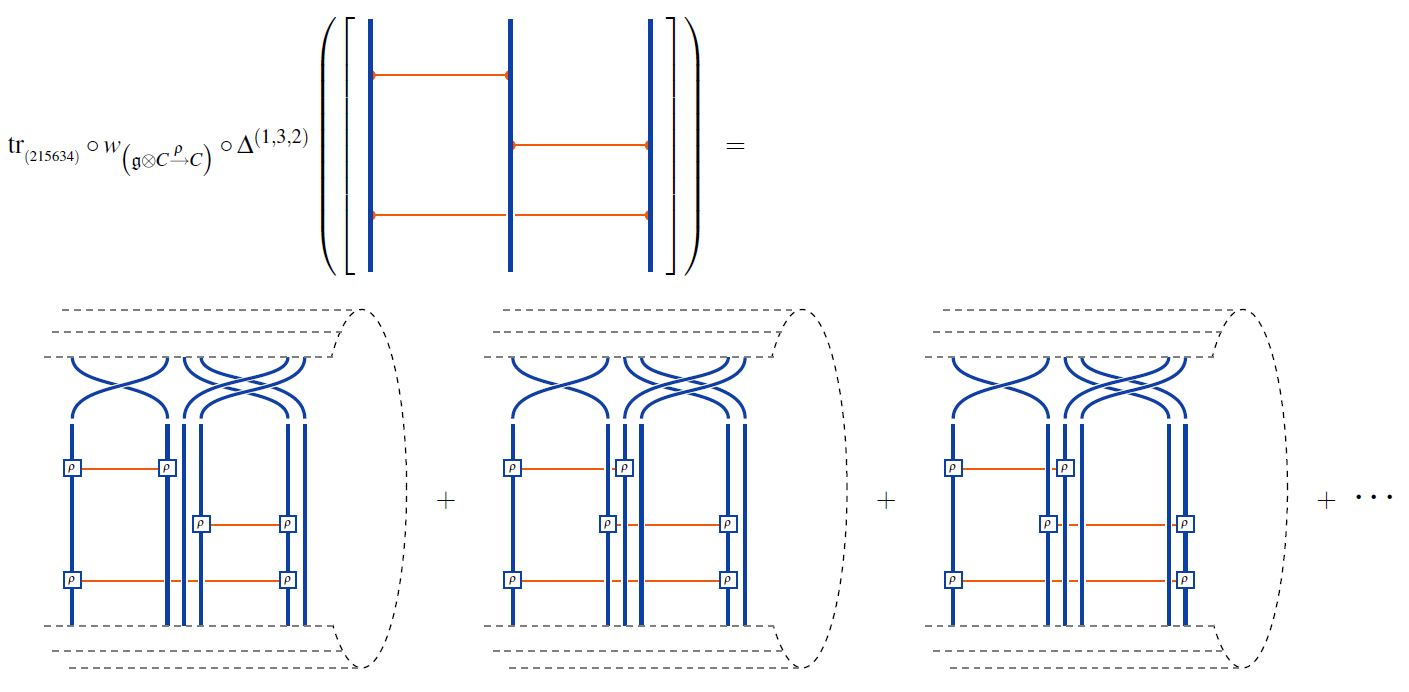graphics from Sati-Schreiber 19c

## Applications

### Chord diagrams as multi-trace observables in the BMN matrix model

The supersymmetric states of the BMN matrix model are temporally constant complex matrices which are complex metric Lie representations $\mathfrak{g} \otimes V \overset{\rho}{\to} V$ of $\mathfrak{g}=$su(2) (interpreted as fuzzy 2-sphere noncommutative geometries of giant gravitons or equivalently as fuzzy funnels of D0-D2 brane bound states).

A fuzzy 2-sphere-rotation invariant multi-trace observable on these supersymmetric states is hence an expression of the following form: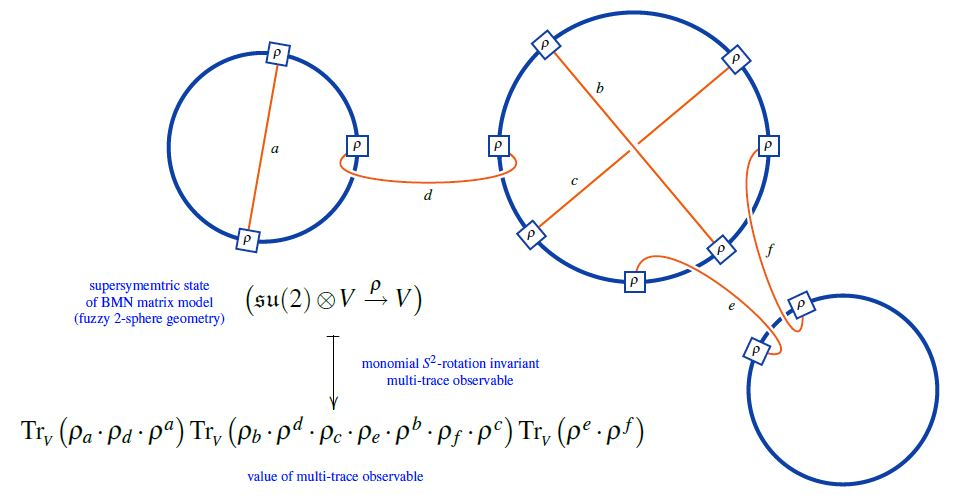Here we are showing the corresponding string diagram/Penrose notation for metric Lie representations, which makes manifest that

1. these multi-trace observables are encoded by Sullivan chord diagrams $D$

2. their value on the supersymmetric states $\mathfrak{su}(2) \otimes V \overset{\rho}{\to}V$ is the evaluation of the corresponding Lie algebra weight system $w_{{}_V}$ on $D$.

Or equivalently, if $\widehat D$ is a horizontal chord diagram whose $\sigma$-permuted closure is $D$ (see here) then the values of the invariant multi-trace observables on the supersymmetric states of the BMN matrix model are the evaluation of $w_{V,\sigma}$ on $\widehat D$, as shown here: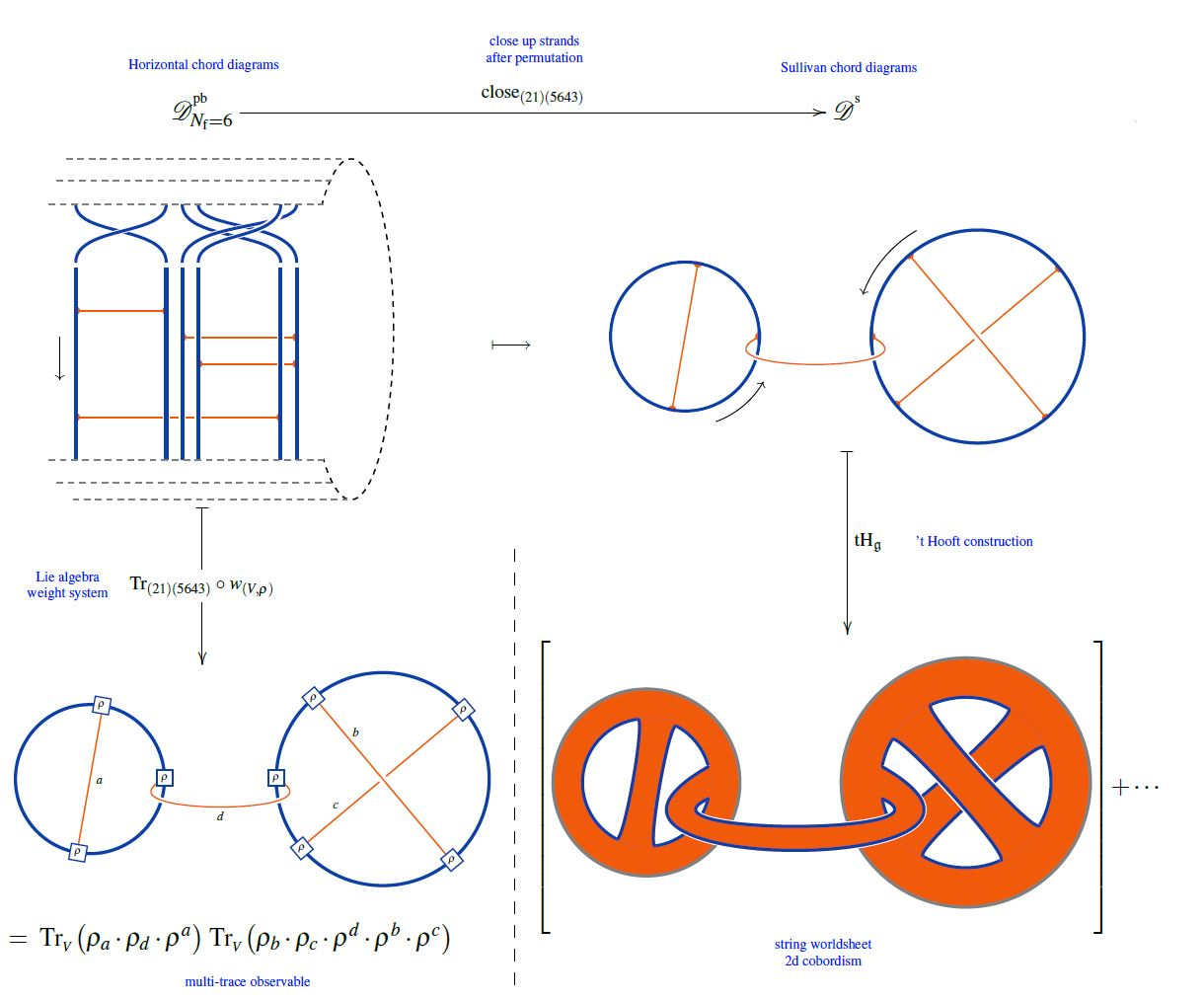But since all horizontal weight systems are partitioned Lie algebra weight systems this way, this identifies supersymmetric states of the BMN matrix model as seen by invariant multi-trace observables as horizontal chord diagrams evaluated in Lie algebra weight systems.

The theorem and its proof is due to: